# Note to Self: Norm of a Gaussian Random Vector

Let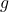be a standard Gaussian vector—that is, a vector populated by independent standard normal random variables. What is the expected length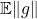of? (Here, and throughout,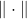denotes the Euclidean norm of a vector.) The length ofis the square root of the sum ofindependent standard normal random variables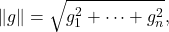which is known as a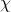random variable withdegrees of freedom. (Not to be confused with a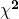random variable!) Its mean value is given by the rather unpleasant formula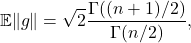where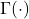is the gamma function. If you are familiar with the definition of the gamma function, the derivation of this formula is not too hard—it follows from a change of variables to-dimensional spherical coordinates.

This formula can be difficult to interpret and use. Fortunately, we have the rather nice bounds

(1)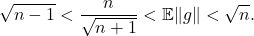This result appears, for example, page 11 of this paper. These bounds show that, for large,is quite close to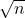. The authors of the paper remark that this inequality can be probed by induction. I had difficulty reproducing the inductive argument for myself. Fortunately, I found a different proof which I thought was very nice, so I thought I would share it here.

Our core tool will be Wendel’s inequality (see (7) in Wendel’s original paper): Forand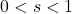, we have

(2)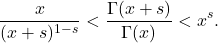Let us first use Wendel’s inequality to prove (1). Indeed, invoke Wendel’s inequality with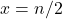and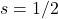and multiply by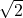to obtain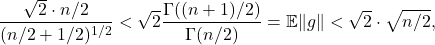which simplifies directly to (1).

Now, let’s prove Wendel’s inequality (2). The key property for us will be the strict log-convexity of the gamma function: For real numbers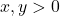and,

(3)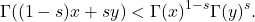We take this property as established and use it to prove Wendel’s inequality. First, use the log-convexity property (3) with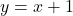to obtain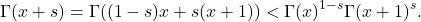Divide by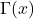and use the property that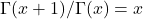to conclude

(4)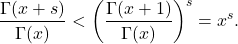This proves the upper bound in Wendel’s inequality (2). To prove the lower bound, invoke the upper bound (4) with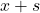in place of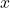and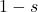in place of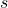to obtain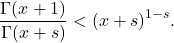Multiplying by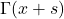, dividing by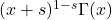, and usingagain yields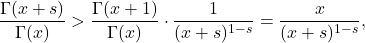finishing the proof of Wendel’s inequality.

Notes. The upper bound in (1) can be proven directly by Lyapunov’s inequality: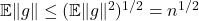, where we use the fact that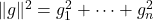is the sum ofrandom variables with mean one. The weaker lower bound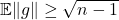follows from a weaker version of Wendel’s inequality, Gautschi’s inequality.

After the initial publication of this post, Sam Buchanan mentioned another proof of the lower boundusing the Gaussian Poincaré inequality. This inequality states that, for a function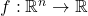,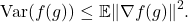To prove the lower bound, set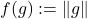which has gradient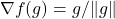. Thus,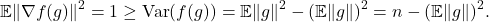Rearrange to obtain.